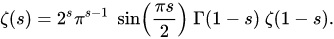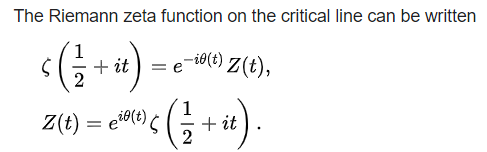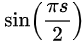### New Odd Numbers Identity and the None-trivial Zeros of Zeta Function

•  Shaimaa said soltan

#### Abstract

This paper is going to introduce a new identity unit circle function for complex plane specific for odd numbers.

Second, we are going to show some properties of these new unit Identity function.

Third, use this new unit Identity function to study the distribution of odd roots for sin term in zeta function but using the new identity function not Euler Identity to explain Riemann conjunction about the critical strip line and the none-trivial zeros along Re(S) = 0.5.

Also, In an Introductory Analysis for the geometric functions Sin and Cos, we will visualize the inverse of geometric function Sin.

Riemann's functional equationThen Zeta function will be zero

1. Atis Zero for any complex number S.
2. If exponential term is zero also when S = S + 0.5 where S is any complex number.This work is licensed under a Creative Commons Attribution 4.0 License.
• ISSN(Print): 1916-9795
• ISSN(Online): 1916-9809
• Started: 2009
• Frequency: bimonthly

### Journal Metrics

• h-index (December 2021): 22
• i10-index (December 2021): 78
• h5-index (December 2021): N/A
• h5-median (December 2021): N/A